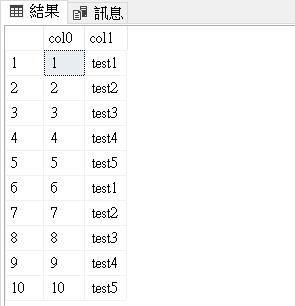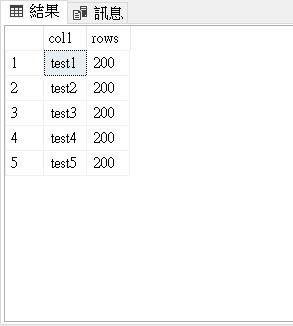### [SQL]無法在 View 裡面使用 MAXRECURSION 的參數

``````with A as
(
select 1 col0, cast('test1' as varchar(5)) col1
union all
select col0+1, cast('test'+cast((col0)%5 +1 as varchar(1)) as varchar(5) )
from A where col0 < 10
)
SELECT * FROM A````````````CREATE VIEW v_Sample1 AS
with A as
(
select 1 col0, cast('test1' as varchar(5)) col1
union all
select col0+1, cast('test'+cast((col0)%5 +1 as varchar(1)) as varchar(5) )
from A where col0 < 1000
)
SELECT * FROM A
GO``````

``````CREATE OR ALTER VIEW v_Sample2 AS
SELECT col1,count(*) rows FROM v_Sample1 where col0 < 101
GROUP BY col1
GO

SELECT * FROM v_Sample2 OPTION (MAXRECURSION 0)
GO``````

``````CREATE OR ALTER Function udf_Sample1()
RETURNS TABLE
AS
RETURN
(
with A as
(
select 1 col0, cast('test1' as varchar(5)) col1
union all
select col0+1, cast('test'+cast((col0)%5 +1 as varchar(1)) as varchar(5) )
from A where col0 < 1000
)
SELECT * FROM A
)
GO

SELECT * FROM udf_Sample1() OPTION (MAXRECURSION 0)
GO``````

``````CREATE OR ALTER Function udf_Sample2()
RETURNS @OUTPUT TABLE ( col0 int, col1 varchar(10) )
AS
BEGIN
with A as
(
select 1 col0, cast('test1' as varchar(5)) col1
union all
select col0+1, cast('test'+cast((col0)%5 +1 as varchar(1)) as varchar(5) )
from A where col0 < 1000
)
INSERT INTO @OUTPUT
SELECT * FROM A OPTION (MAXRECURSION 0);
RETURN
END
GO

SELECT col1,count(*) rows
FROM udf_Sample2()
GROUP BY col1
ORDER BY col1
GO``````